# 数据库内核杂谈（八）：数据库优化器（下）## 启发式算法 (Heuristic approach)

4^n 的搜索空间实在太大了，首先要做的就是，减小搜索空间。数据库的先贤们，想到了应用启发式算法 (heuristic approach) 来降低搜素空间。比如，最早的 Volcano Optimizer 里，就提出了对于 Join-order，只考虑 left-deep-tree，即所有的右子树必须是一个实体表。可能解释起来不太直观，对应下面三张不同的 join-order 的树形结构，就一目了然了。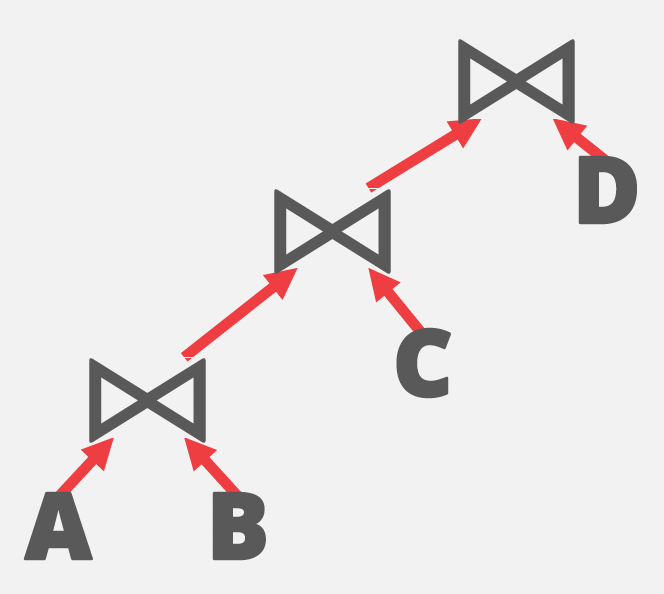left-deep-tree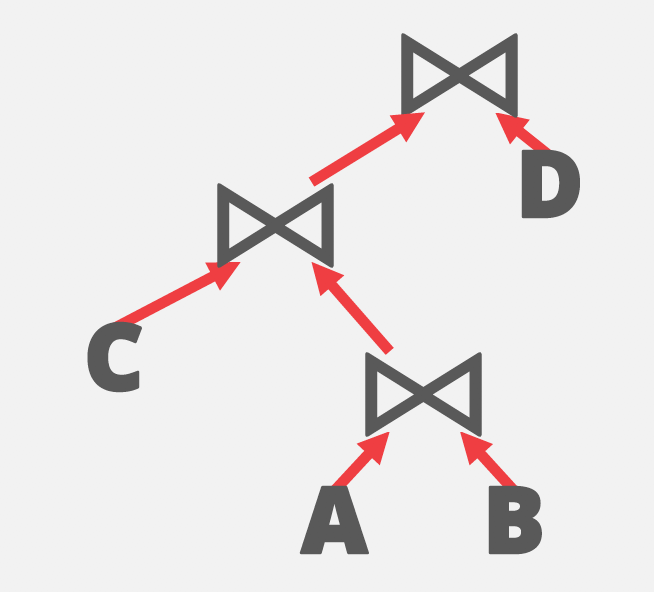bushy-tree 1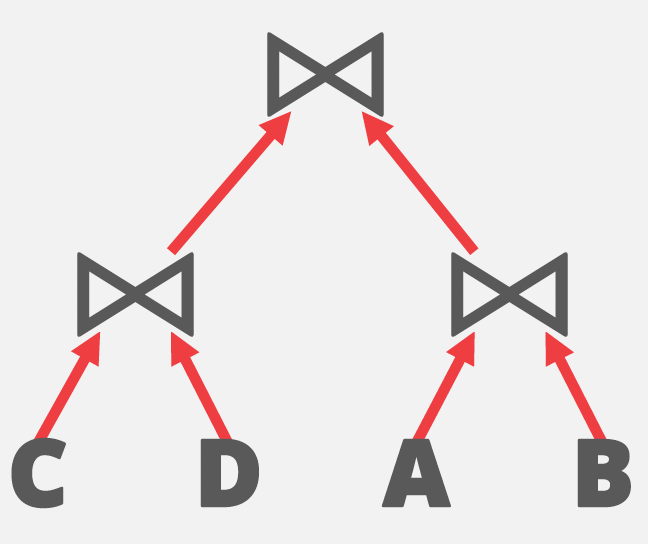bushy-tree 2

## Cardinality Estimation

Cardinality estimation，就是用来预测单个表的 selection cardinality 和 2 个表的 join cardinality 的技术。首先，简单介绍一些术语。

Selection cardinality: SC(P, R)

SELECT * FROM student WHERE major = 'CS';

SC(P, R) = N_R * SEL(P, R)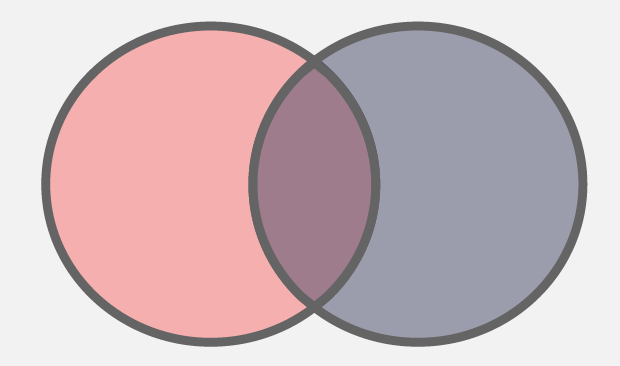disjunction

SEL * FROM R1, R2 WHERE R1.a = R2.a;

JC(R1, R2, R1.a = R2.a) = N_R * N_S / max(V(a, R), V(a, S))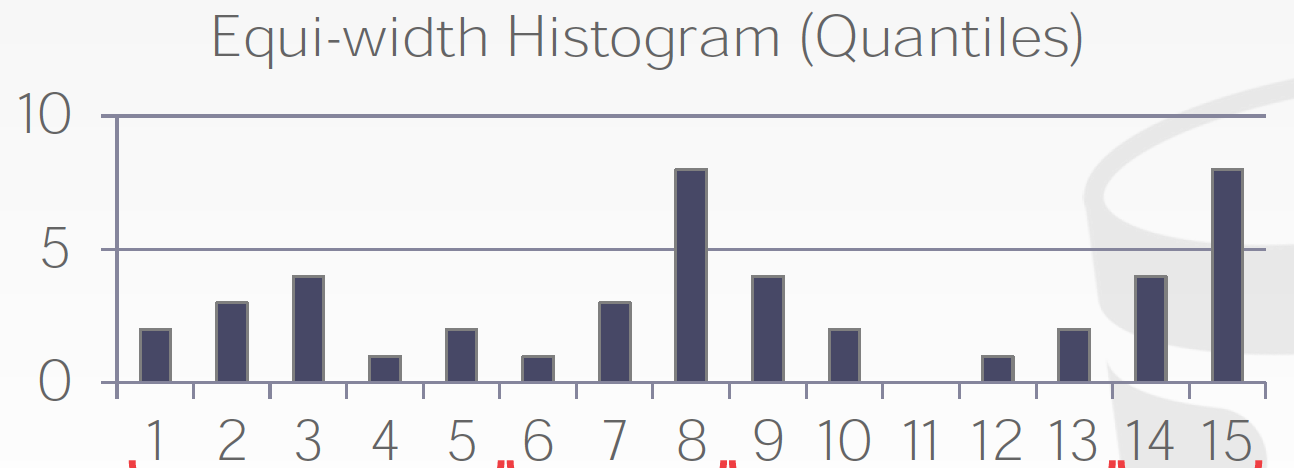Histogram example

Histogram 通过存储更多的信息来统计更精确的数值分布。但很多情况下，统计并不需要那么精确。工程方面要在使用资源和准确性里找平衡。后来，又有大牛提出了 HyperLogLog ，是一种占用资源少，但能给出较为准确的近似结果的 cardinality estimator。

Cardinality estimation 仅仅是帮助决定了 join ordering，相当于 logical operator tree 上，不同的表分别应该放在哪个位置。但对于每个 logical operator，最后还需要变换成 physical operator (物理算子) 才算完成最终的优化。如，对与 TableScan 这个逻辑算子，它对应的 physical operator 有 SequentialScan 和 IndexScan，应该用哪个？ 对于 JoinOperator，到底是用 NestedLoopJoin, SortMergeJoin，还是 HashJoin 呢？下面，我们介绍第二个关键技术。

## Cost Model

Cost Model 的主要思想是，对于每一个 Physical operator，根据输入输出的 cardinality，会被赋值一个 cost(代价)。这个 cost，通常情况下可以认为是执行这个 operator 需要的时间，当然也可以是计算资源。那如何计算这个 cost 呢？对于每个 operator，都有一个 cost formula(公式)，这个公式根据输入输出的信息，最后能计算出这个 operator 的 cost 值。

Cost = NumRowsInTable * RowWidth * SEQ_SCAN_UNIT_COST

Cost = SelectionCardinality * RowWidth * INDEX_SCAN_UNIT_COST

SELECT * FROM student WHERE major = 'CS';

SequentialScan Cost = 10000 * 10 * 1 = 100000 IndexScan Cost = 500 * 10 * 100 = 500000

SequentialScan Cost = 10000 * 10 * 1 = 100000 IndexScan Cost = 10 * 10 * 100 = 10000